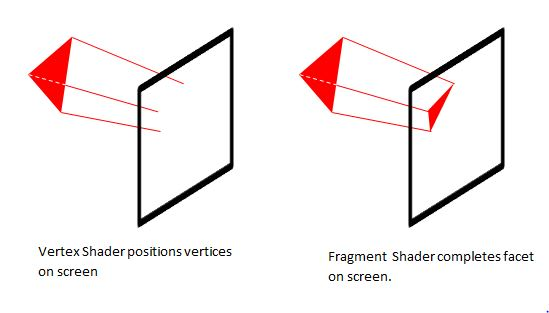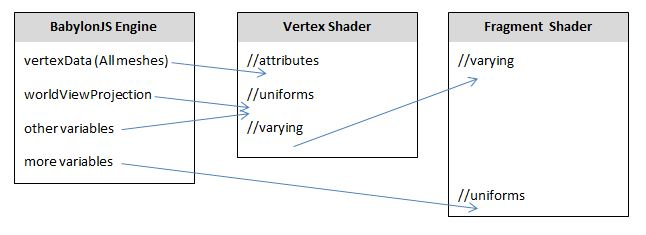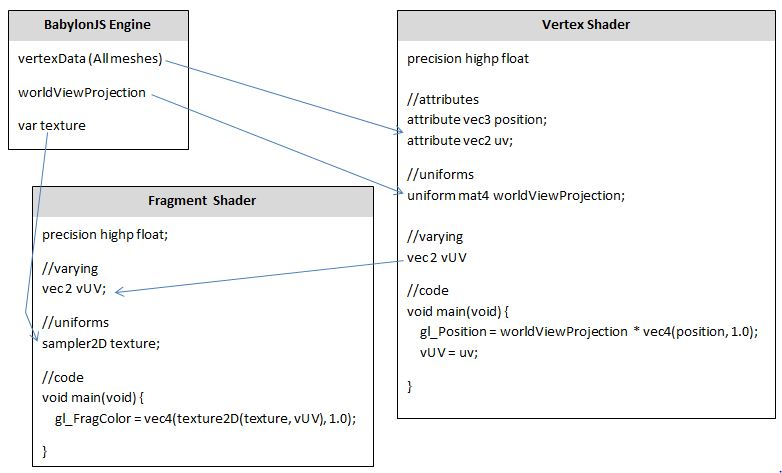# Introduction to Shaders in BabylonJS

A shader is a program processed by the Graphical Processing Unit (GPU) to produce a screen image by manipulating data to produce individual pixels. The GPU is optimised, through parallel processing, to deal with these thousand of operations in an extremely fast way.

## An Overview

### Basic

To produce a BabylonJS scene, code is written in Javascript which the BabylonJS Engine processes and displays the result on screen. The scene can alter through changes to the meshes, the lights or camera position. To show possible changes in a timely way the screen display (frame) is re-drawn up to 60 frames per second.

Simplifying, the process is

• Scene Code is processed in the CPU by the BJS Engine Code to produce a Virtual 3D Model
• Virtual 3D Model is processed in the CPU by the BJS Engine Code to produce Shader GPU Code
• Shader GPU Code is processed by GPU to produce screen image.

For example the BabylonJS Engine takes this code

``````var box = BABYLON.MeshBuilder.CreateBox("box", {}, scene);
``````

and turns it into vertex data including positions, colors and normals

The BabylonJS Engine creates the shader code for this data and is passed to the GPU.

### Custom

Much more than this as well as Scene Code you can write your own user Shader Code so that the process becomes

• Scene Code is processed in the CPU by the BJS Engine Code to produce a Virtual 3D Model
• Virtual 3D Model and User Shader Code is processed in the CPU by the BJS Engine Code to produce the Shader GPU Code
• Shader GPU Code is processed by GPU to produce the screen image.

## Types of Shader

Shaders are written in Graphics Library Shader Language (GLSL) and come in two parts.

1. Vertex Shader - this takes the data for each vetex and determines where on the screen the pixel for it will be displayed and its color.

2. Fragment Shader - this uses data from the Vertex Shader to determine the position and colour of the pixels to represent each facet of the mesh.

Fragement Shaders are sometimes refered to as Pixel Shaders.## Passing Variables

The vertex data for position, normal and uv coordinates are passed to the Vertex Shader as variables of category attribute. User data can be passed to both the Vertex Shader and the Fragment Shader as variables of category uniform. Data can be passed from the Vertex Shader to the Fragment Shader with variables of category varying.

A vital uniform variable to declare in the Vertex Shader is worldViewProjection as the BabylonJS Engine uses this to pass scene 3D - 2D projection data to the Vertex Shader.## Variable types

All variables used in both shaders must be given a type and any numbers assigned to the variable must be consistent with its type.

For example

``````int n = 2;
float r = 2.0;
``````

The following example with throw an error

``````float r = 2;
``````

Some examples of types are

vec2 ------ a two dimensional vector of floating-point numbers
vec3 ------ a three dimensional vector of floating-point numbers
mat4 ------ a matrix with 4 columns and 4 rows floating-point numbers
sampler2D - a 2D texture image

Since vertex positions need to be as accurate as possible all floating-point numbers should be set as having high precision. This is done at the start of the code for each shader using -

``````precision highp float
``````

## Built In Variables

The GLSL language has a number of built in variables. Two are vital to the operation of the two shaders and are always neceesary.

gl_Position which provides positional data for screen coordinates

gl_FragColor which provides colour data for the representation of a facet on screen.

## Functions

Functions needed to be typed as do their parameters and have the form

``````float NAME(typed parameters) {
*code*
}
``````

## Running Shader Code

Both the Vertex and the Fragment Shader are run from a function which must be called main and be of type void since it returns no result. It must also must type the empty parameter list as void.

``````void main(void) {
*code*
}
``````## Putting Shader Code in BabylonJS

Here are four ways of putting shader code into your scene: